# Superconducting Gap Equation for the Negative-U Hubbard Model

## Taylor L. Hughes June 2, 2008

### (Submitted as coursework for Appied Physics 373, Stanford University, Spring 2008)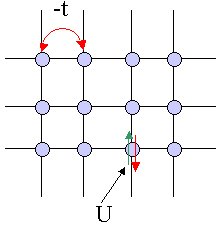Fig. 1: Hubbard model on a square lattice with hopping -t and onsite energy U.

In this page we will study a the mean-field theory of the Hubbard model. The half-filled Hubbard model with U>0 is written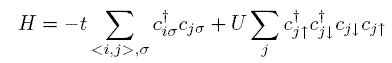At half-filling this model, for any value of U>0 is unstable toward the formation of anti-ferromagnetic order. The anti-ferromagnetic order parameters are given by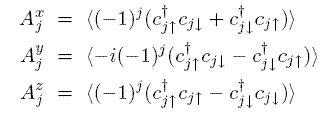We want to study a different set of phases, related to these by a cannonical transformation. We want to apply a transformation to the Hubbard model which converts the positive U to a negative U and exploit what we already know about the anti-ferromagnetic order to study superconductivity in the transformed model. The transformation we will apply is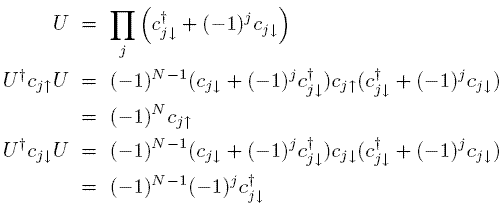where N is the number of lattice sites. Now we can transform the full Hamiltonian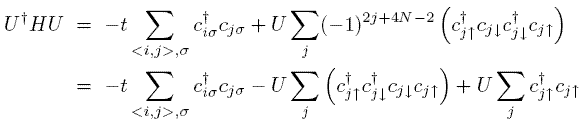This transformation effectively flips the sign of U. The third term in the transformed Hamiltonian can effectively be treated like a constant and dropped when the number of particles is large. In this case the amount of particle fluctuations is suppressed compared to the number of particles and so this contribution becomes negligible. This transformation gains us something extra as well. We can transform the anti-ferromagnetic order parameters to get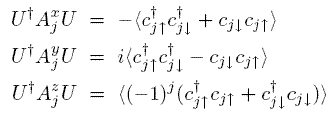The first two order parameters are proportional to the real and imaginary parts of a superconducting order parameter, and the third is a crystallization order parameter whose non-zero expectation value would indicate an inhomogeneous charge ordering.

## The Hartree-Fock Hamiltonian and the Gap Equation

Now we will construct a Hartree-Fock Hamiltonian for the superconducting order parameter from a mean-field theory argument. First use the following trivial rewriting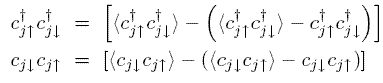After being rewritten these terms look like a mean-field expectation value plus a fluctuation term. We will substitute these forms into the interacting part of the Hamiltonian and only keep terms up to the leading order in the fluctuations. The interacting part of the Hamiltonian becomes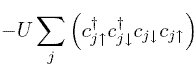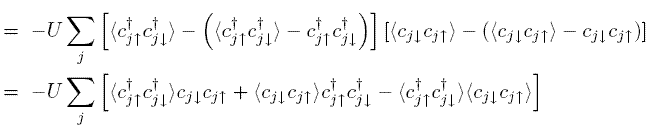Now we can write down a mean-field ansatz and the Hartree-Fock Hamiltonian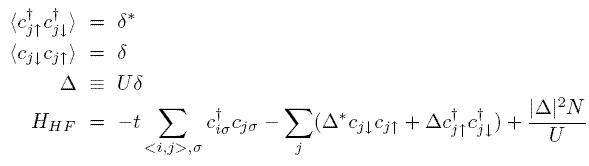We want to diagonalize the Hamiltonian so first we Fourier transform and then we use a trick to write it in the form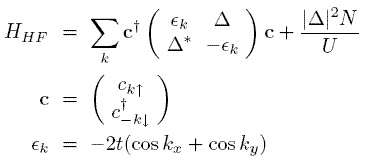where I have set the lattice constant to 1. The k-dependent part of the spectrum of this Hamiltonian is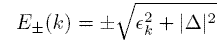although what the spectrum means is subtle because the eigenfunctions are combinations of particles and holes.

At half-filling all the negative energy states are filled, and if we assume an even number of sites, effectively all the fermions are paired so there are no quasi-particles in the upper band. We can define an energy functional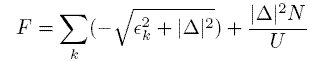Now we want to demand that the true value of the order parameter is a stationary point of this functional. This will give us the self-consistent gap equation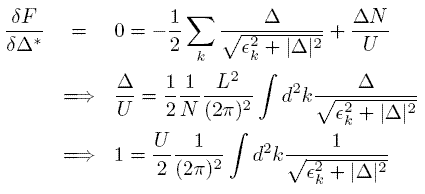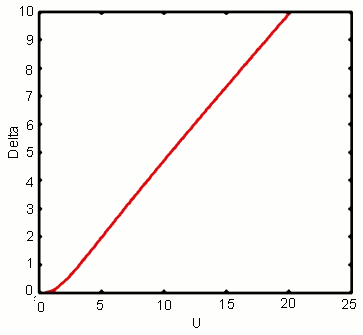Fig. 2: Spin density wave gap Δ versus U.

We can simply integrate the right hand side to show that a solution always exists. That is, given any U there will be a corresponding Δ. The easiest way to calculate is to input a gap Δ calculate the integral, and see what value of U satisfies the gap equation. One can simply invert the functional dependence to get Δ as a function of U. See the figure below.

This figure shows that, at half-filling, in the negative U Hubbard model, the system is absolutely unstable toward the formation of superconducting order. We could have also picked the charge-ordering parameter and shown the same thing. The system could pick either type of order or some pathological combination although the physics of that type of phase is not straightforward.

## Source Code

The source code for all of the calculations done in this work can be found here. Typical execution times for a grid size of 1800x1800 momentum points and for many values of Δ were a few minutes.

© 2008 T. L. Hughes. The author grants permission to copy, distribute and display this work in unaltered form, with attribution to the author, for noncommercial purposes only. All other rights, including commercial rights, are reserved to the author.